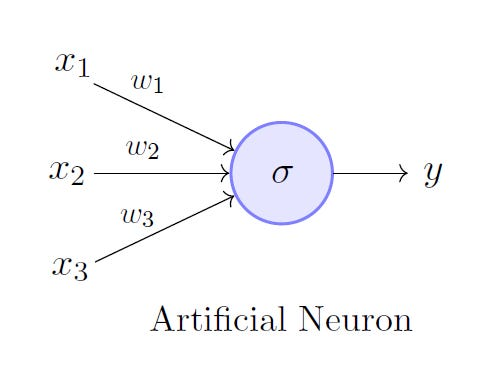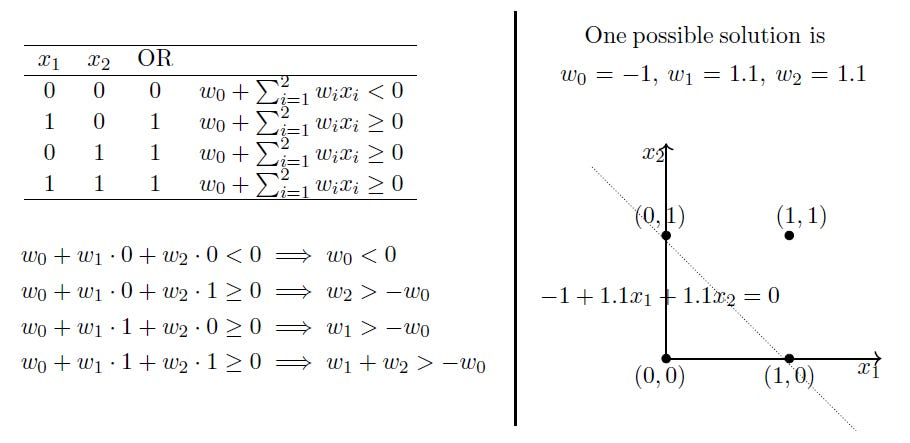# Perceptron: The Artificial Neuron

The most fundamental unit of a deep neural network is called an artificial neuron. Frank Rosenblatt, an American psychologist, proposed the classical perceptron model (1958). Further refined and carefully analyzed by Minsky and Papert (1969) — their model is referred to as the perceptron model. This is a follow-up post to my previous post on McCulloch-Pitts neuron, I suggest you at least quickly skim through it to better appreciate the Minsky-Papert contributions.

Note: The concept, the content, and the structure of this article was inspired by the awesome lectures and the material offered by Prof. Mitesh M. Khapra on NPTEL’s Deep Learning course. Check it out!

### Perceptron

A perceptron is a more general computational model than McCulloch-Pitts neuron. It overcomes the limitations of the M-P neuron by introducing the concept of numerical weights (a measure of importance) for inputs, and a mechanism for learning those weights. Inputs are no longer limited to boolean values like in the case of an M-P neuron, it supports real inputs as well which makes it more useful and generalized.

Now this is very similar to an M-P neuron but we take a weighted sum of the inputs and set the output as one only when the sum is more than an arbitrary threshold (theta). However, according to the convention, instead of hand coding the thresholding parameter thetha, we add it as one of the inputs, with the weight –theta like shown below, which makes it learn-able (more on this in my next post — Perceptron Learning Algorithm).

Consider the task of predicting whether I would watch a random game of football on TV or not (the same example from my M-P neuron post) using the behavioral data available. And lets assume my decision is solely dependent on 3 binary inputs (binary for simplicity).

Here, w_0 is called the bias because it represents the prior (prejudice). A football freak may have a very low threshold and may watch any football game irrespective of the league, club or importance of the game [theta = 0]. On the other hand, a selective viewer like me may only watch a football game that is a premier league game, featuring Man United game and is not friendly [theta = 2]. The point is, the weights and the bias will depend on the data (my viewing history in this case).

Based on my data, if needed the model may have to give a lot of importance (high weight) to the isManUnitedPlaying input and penalize weights of other inputs.

### Perceptron vs McCulloch-Pitts Neuron

What kind of functions can be implemented using a perceptron? How different is it from McCulloch-Pitts neurons?

From the equations, it is clear that even a perceptron separates the input space into two halves, positive and negative. All the inputs that produce an output 1 lie on one side (positive half space) and all the inputs that produce an output 0 lie on the other side (negative half space).

In other words, a single perceptron can only be used to implement linearly separable functions, just like the M-P neuron. Then what is the difference? Why do we claim that the perceptron is an updated version of an M-P neuron? Here, the weights, including the threshold can be learned and the inputs can be real values.

### Example: OR Function

Just revisiting the good old OR function the perceptron way.Feel free to try solving the linear equations for other possible solutions.

The above ‘possible solution’ was obtained by solving the linear system of equations on the left. It is clear that the solution separates the input space into two spaces, negative and positive half spaces. It works!

The XOR Affair

In the book published by Minsky and Papert in 1969, the authors implied that, since a single artificial neuron is incapable of implementing some functions such as the XOR logical function, larger networks also have similar limitations, and therefore should be dropped. Later research on three-layered perceptrons showed how to implement such functions, therefore saving the technique from obliteration.

Wikipedia

### Conclusion

In this post, we looked at a perceptron, the fundamental unit of deep neural networks. We also showed with an example how a perceptron, in contrast with the McCulloch-Pitts neuron, is more generalized and overcomes some of the pertaining limitations at the time.

In my next post, we will closely look at the famous Perceptron Learning Algorithm and try and get an intuition of why it works, without getting into any of the complex proofs, along with an implementation of the algorithm in Python from scratch.

Thank you for reading the article.
Live and let live!
A

Source: Deep Learning on Medium